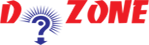﻿ How to find the Square Root?## Python Program to Find the Square Root

This Python program describes that how to find the square root of a number by using exponent operator and cmath module. The prerequisites to understand the program are Python Input, Output and Import, Data Types and Operators.

``````
Example:
For Positive numbers using exponent **
# Python Program to calculate the square root

# Note: change this value for a different result

num = 8
# uncomment to take the input from the user
#num = float(input('Enter a number: '))
num_sqrt = num ** 0.5
print('The square root of %0.3f is %0.3f'%(num ,num_sqrt))

Output

The square root of 8.000 is 2.828
```
```

Conclusion: Here you can see that the number is stored in num to find the square root using the exponent ** operator. this python program works for all positive real numbers.

For negative or complex number, you can copy the python code as given below:

``````
Example: For real or complex numbers using cmath module
# Find square root of real or complex numbers
# Import the complex math module
import cmath

# change this value for a different result
num = 1+2j

# uncommment to take input from the user
#num = eval(input('Enter a number: '))
num_sqrt = cmath.sqrt(num)
print('The square root of {0} is {1:0.3f}+{2:0.3f}j'.format(num ,num_sqrt.real,num_sqrt.imag))
Output
The square root of (1+2j) is 1.272+0.786j
```
```

Conclusion: Here, we use the sqrt() function in the complex math module. In this program, eval() function is used instead of float() to convert complex number as well.

## Other Python Programs

PythonTraining, 258-FF , Katewa Nagar, New Sanganer Rd, Jaipur, 302019